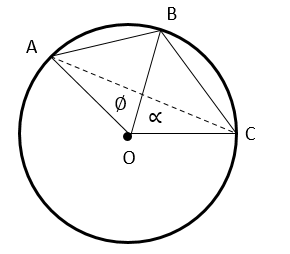# IPMAT Question Paper 2019 | IPM Indore Quants

###### IPMAT Sample Paper | IPMAT Question Paper | Question 33

IPMAT 2019 Question Paper IPM Indore Quantitative Ability. Solve questions from IPMAT 2019 Question Paper from IPM Indore and check the solutions to get adequate practice. The best way to ace IPMAT is by solving IPMAT Question Paper. To solve other IPMAT Sample papers, go here:IPM Sample Paper

Question 33: On a circular path of radius 6 m a boy starts from a point A on the circumference and walks along a chord AB of length 3 m. He then walks along another chord BC of length 2 m to reach point C. The point B lies on the minor arc AC. The distance between point C from point A is

1. $$frac{\sqrt{15} + \sqrt{35}}{2}\\$ m 2. 8 m 3. √13 m 4. 6 m ## Best CAT Online Coaching Try upto 40 hours for free Learn from the best! #### 2IIM : Best Online CAT Coaching. ### Video Explanation ## Best CAT Coaching in Chennai #### CAT Coaching in Chennai - CAT 2022Limited Seats Available - Register Now! ### Explanatory Answer We are given that, OA = OB = OC = 6m. AB = 3m. BC = 2m. Let’s say angle AOB = ∅ and angle BOC = ∝. Cos ∅ = $\frac{AO^{2} + BO^{2} - AB^{2}}{2.𝐴𝑂.𝐵𝑂}\\$ = $\frac{6^{2} + 6^{2} - 3^{2}}{2.6.6}\\$ = $\frac{63}{72}\\$ = $\frac{7}{8}\\$ Sin ∅ = $\sqrt{1 − cos^{2} ∅}\\$ = $\sqrt{1 −$$frac{7}{8}$^{2}}$\$ = $\sqrt{\frac{64 - 49}{64}}\\$ = $\sqrt{\frac{15}{64}}\\$ = $\frac{\sqrt{15}}{8}\\$Similarly Cos ∝ = $\frac{CO^{2} + BO^{2} - AC^{2}}{2.𝐴𝑂.C𝑂}\\$= $\frac{6^{2} + 6^{2} - 2^{2}}{2.6.6}\\$= $\frac{68}{72}\\$ = $\frac{17}{18}\\$ Sin ∝ = $\sqrt{1 − cos^{2} ∝}\\$ = $\sqrt{1 −$$frac{17}{18}$^{2}}$\$ = $\sqrt{\frac{35}{324}}\\$ = $\frac{\sqrt{35}}{18}\\$ Cos$∅ + ∝) = Cos ∅ Cos ∝ - Sin ∅ Sin ∝
= $$frac{7}{8}\\$ . $\frac{17}{18}\\$ - $\frac{\sqrt{15}}{8}\\$ . $\frac{\sqrt{35}}{18}\\$ = $\frac{119 - 5\sqrt{21}}{8.18}\\$ Also, Cos$∅ + ∝) = $$frac{AO^{2} + CO^{2} - AC^{2}}{2.𝐴𝑂.C𝑂}\\$ =$\frac{6^{2} + 6^{2} - AC^{2}}{2.6.6}\\$ = $\frac{72 - AC^{2}}{72}\\$ $\frac{72 - AC^{2}}{72}\\$ = $\frac{119 - 5\sqrt{21}}{8*18}\\$ AC2= 72 - $\frac{72$119 - 5$sqrt{21}$}{8*18}$\$ = $\frac{72*8*18 - 72*119 + 72*5\sqrt{21}}{8*18}\\$ = $\frac{25 + 5\sqrt{21}}{2}\\$ Going by the options if AC = $\frac{\sqrt{15} + \sqrt{35}}{2}\\$ AC2= $\frac{$$sqrt{15} + \sqrt{35}$^{2}}{4}$\$ = $\frac{15 + 35 + 2\sqrt{15*35}}{4}\\$ AC2= $\frac{50 + 10\sqrt{21}}{4}\\$ AC2= $\frac{25 + 5\sqrt{21}}{2}\\$, which concurs with our calculated value of AC2. Therefore, The distance between C and A is $\frac{\sqrt{15} + \sqrt{35}}{2}\\$ m. The question is"On a circular path of radius 6 m a boy starts from a point A on the circumference and walks along a chord AB of length 3 m. He then walks along another chord BC of length 2 m to reach point C. The point B lies on the minor arc AC. The distance between point C from point A is" ##### Hence, the answer is $\frac{\sqrt{15} + \sqrt{35}}{2}\\$ m Choice A is the correct answer ###### Best Indore IPM & Rohtak IPM CoachingSignup and sample 9 full classes for free. Register now! ###### Already have an Account? ##### Where is 2IIM located? 2IIM Online CAT Coaching A Fermat Education Initiative, 58/16, Indira Gandhi Street, Kaveri Rangan Nagar, Saligramam, Chennai 600 093 ##### How to reach 2IIM? Mobile:$91) 99626 48484 / 94459 38484
WhatsApp: WhatsApp Now
Email: info@2iim.com